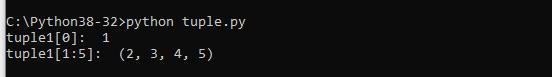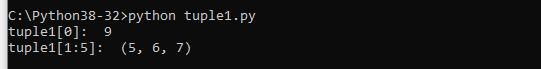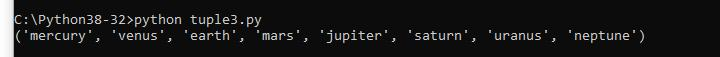Home » Python Tuples : the basics

# Python Tuples : the basics

A tuple is a collection which is ordered and unchangeable. This means you can not add an item to a tuple and you cannot remove an item from a tuple. If you do this it will raise an error

Creating a tuple is very easy all you need to do is put different comma separated values. AN option which I always use is to put these values between parentheses also.

```tuple1 = (‘physics’, ‘chemistry’, 1997, 2000);
tuple2 = (1, 2, 3, 4, 5, 6, 7 );
tuple3 = “mercury”, “venus”, “earth”, “mars”;```

You can createrr an empty tuple by simply writing two parentheses with no values

`tuple1 = ();`

You can also create a tuple which only contains one value by simple writing a tuple with one value and then a comma

`tuple1 = (2020,);`

tuple indices start at 0,

As you can see tuples are very similar to lists

### Accessing Tuple Items

You can access tuple items by referring to the index number, inside square brackets. Lets look at 2 examples

```#!/usr/bin/python
​
tuple1 = (1, 2, 3, 4, 5, 6, 7,8,9 );
print ("tuple1: ", tuple1);
print ("tuple1[1:5]: ", tuple1[1:5]);```

When this executed this you will see something like thisYou can also use negative indexing which means you start from the end, -1 refers to the last item. Lets look at some examples

```#!/usr/bin/python

tuple1 = (1, 2, 3, 4, 5, 6, 7,8,9 );
print ("tuple1: ", tuple1[-1]);
print ("tuple1[1:5]: ", tuple1[-5:-2]);```

You will see this### More Tuple functionality

We will now look at getting the length of a tuple, check if an item is in a tuple, looping through all of the items in a tuple

To determine if an item is present in a tuple you can use the in keyword.
To determine how many items a tuple has you can use the len() method.
You can use a for loop to loop through all of the items that are present in a tuple and also print them out.

Lets look at an example

```#!/usr/bin/python

tuple1 = ("mercury", "venus", "earth", "mars", "jupiter", "saturn", "uranus", "neptune")
print(len(tuple1))

if "jupiter" in tuple1:
print("Jupiter is in the tuple")

for x in tuple1:
print(x)```

When you run this you should see the following outputYou can also join two tuples. lets see an example of this

```#!/usr/bin/python

tuple1 = ("mercury", "venus", "earth", "mars")
tuple2 = ("jupiter", "saturn", "uranus", "neptune")

tuple3 = tuple1 + tuple2
print(tuple3)```

When you run this you should see the following output0 comment

#### You may also like

This website uses cookies to improve your experience. We'll assume you're ok with this, but you can opt-out if you wish. Accept Read More